ELEMENTARY STUDY OF LORENTZ' TRANSFORMATIONS

Through an original, purely mathematical and very elementary, study, I will demonstrate:

That Lorentz' formulas are interpretable in the classical space-time (euclidian space-time),
That they have very fundamental natural mechanical representations,
That these formulas do not exclude Galilean transformations ,
That speeds greater than c , c limit speed in Lorentz' formulas, are not excluded, by these
transformations.
That the Einstein's principle of relativity is not the consequence of these transformations.

Beginning of the study
As a simplification the formulas are studied here only in a one dimensional space .

Let S an axis Ox , S1 an axis O1x1
S1 is parallel to S and S1 slide along Ox at a constant speed, v ,
v verifying -c < v < c , c a given positive constant.
This means that the motion of O1, origin of S1, is determined in S, by the equation x = vt + xo .
By an adequate choice of the origin O of S , we can always have xo=0 .
Therefore we will take the equation x = vt  as equation of the motion of O1 , relatively to S .

If E is an event of coordinates (x ,t ) in S , then the coordinates (x1 ,t1 ) of E in S1 are,

by définition of Lorentz' transformations :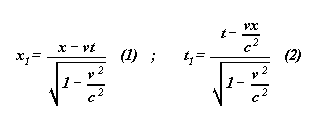Given the two systems S and S1 , one says that one passes from S to S1 by the transformations of Lorentz of constant c .

In the next, one puts: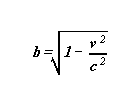b is a constant dépendant only of v , b verifying :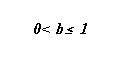( c being unchanged )

page 1

Another expression of t1

From (1) we have : x = vt + bx1 .
If we replace x by this expression in (2) , we obtain :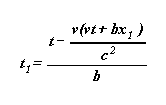Otherwise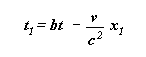this last formula brings out :

1.  The slowing of clocks of S1 , slowing proportional to the multiplicative factor b ,
2.  The phasing-out of clocks of S1 , phasing-out given by the additive term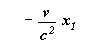,
term proportional to x1 .

And more over, the formula x = vt + bx1 ,compared to the Galilean's transformation
x = vt + x1 , brings out the shortening of lengths of S1 in the Ox direction, shortening always proportional to the multiplicative factor b .

In the following, I will represent systems S and S1 , giving  c and v small numerical values that allow drawing very explicit figures.
These never seen figures will allow us to catch the full meaning of  Lorentz' transformations thoroughly.

Representation of S and S1 in the particular case where :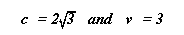With these values one has   c2 = 12 and b = 1/2 ,

that give for x1 and t1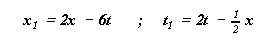Otherwise :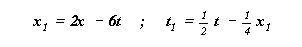The unit of length in S is considered to be equal to 2 centimeters .
These various values enable convenient vizualizing of  S and S1 systems at different times t of S . (See axis of figure 1)
The different drawings of the figure 1 are obtained in the following manner.

For drawing 1, one has  t=0 , and gives x all the values possible in the formulas :

x1 = 2x - 6t ; t1 = 2t - 1/2 x .
With t = 0 , one has therefore : x1 = 2x  and  t1 = - 1/2 x

For example, when  x = - 1 , one has : x1 = -2 and t1 = 1/2 .
This mean that the point of S1 in coincidence with the point of S of abscissa - 1 ,
(at the date t = 0 of S ) has for abscissa x1 = -2 , and that the clock of S1 that is found on this point,
indicates + 1/2  ( +1/2h to meaning that the number read is a time. h for hour) .

Page 2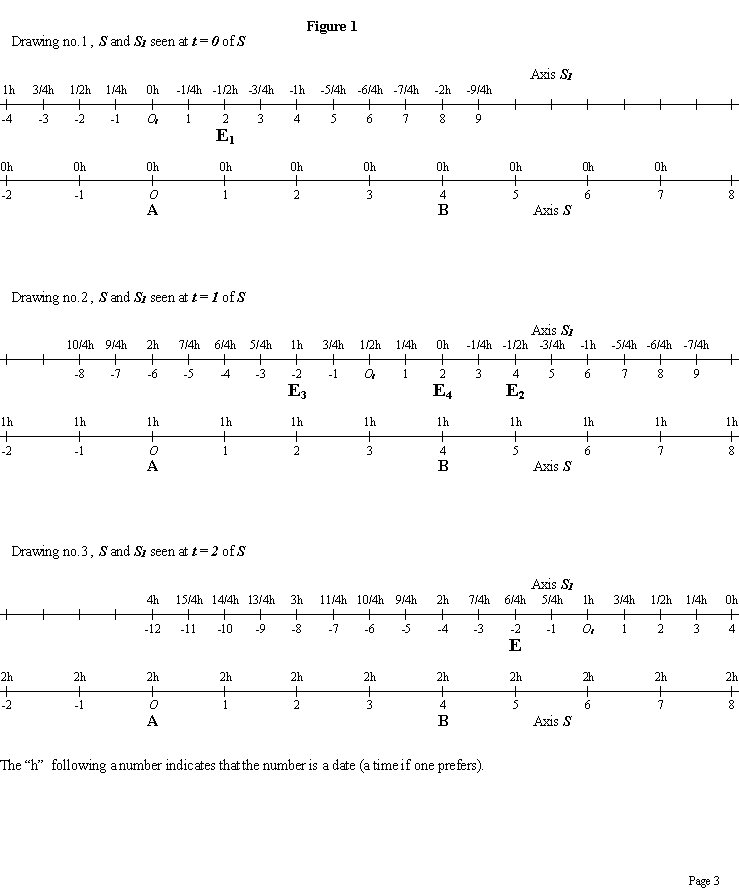Similarly for x = 1/2 (always with t = 0 ) , one obtains x1 = 1 and t1 = - 1/4 ( - 1/4h ) .
The point of S1 in coincidence with the point of S of abscissa +1/2 (in S ) , has for abscissa 1
(in S1) , and the clock of S1 that is found on this point indicates - 1/4 (- 1/4h ) .

Similarly for all the other values of x, always with t = 0 , which gives the system
S1 seen from S at the date t = 0 of S. (See drawing 1 of figure 1)

To obtain the second drawing, one takes t =1 , that gives x1 = 2x - 6 and t1 = 2 - 1/2 x , and one makes x
vary as previously, which gives the system S1 seen from S at the date t = 1 of S .

One proceeds similarly to obtain drawing 3 by taking t = 2 (in S) , which gives
x1 = 2x - 12 and t1 = 4 - 1/2 x .
In the drawings, the only times which are indicated are given by clocks placed on integer abscissas of systems.

The drawings of figure1 lead to the following remaks:

1. Units of length of S1 (in the direction of the speed) are constant, and smaller than the units of length of S .
In the drawings of figure1, the unit of S1 (in the direction of the speed) is half that of S .

2. In S1 , on each point, there is a clock, clock that is used to date events that happen in this point of S1 .
When in S1 , an event happens in the point of abscissa x1 of S1 , the date in S1 of this event is given by
the clock of S1 staying on x1 , and by this one only. That is entirely comparable to terrestrial time zones.
An event taking place in Paris is dated by the Paris time zone, and not by that of Moscow.

Example: On the first drawing (figure 1), E1 represents any event (an explosion for example).

E1 arise in S at the point of abscissa x = 1 , and the clock of S that is found at this point shows then
the date t = 0 . The coordinates of this event are in S : x = 1 and t = 0 .

This same event arise in S1 at the point of abscissa x1 = 2 , and at the date t1 = - 1/2 , date gived by
the clock of S1  placed at the point of abscissa x1 = 2 of S1 ( see, figure 1).

3. Simultaneous events in one system are no longer simultaneous in the other.

Let me first define what are two simultaneous events in a system.

Two events (two explosions, for example) are simultaneous for a system, if, and only if the dates given by
the two clocks of the system that are found in the places (at the core) of these events, indicate the same
numbers (the same time, the same date).

Example: The event E1 of abscissa +2 in S1 and of date - 1/2 always in S1
(an event indicated on the first drawing of figure 1), and the event E2 of abscissa + 4 in S1 and
of date - 1/2 , always in S1 (an event marked on the second drawing of figure 1) ,
are simultaneous relatively to S1 .

One notices that the coordinates of E1 in S are x =1 and t = 0 , and that the coordinates of
E2 in S are x = 5 and t = 1 . E1 and E2 are not simultaneous in S .

Similarly, two simultaneous events in S , not having the same abscissa, are not simultaneous in S 1 .
Example:
On the drawing no. 2 of figure1, the event E3 has for coordinates in S : x = 2 and t = 1 .
The event E4 has for coordinates in S : x = 4 and t = 1 . E3 and E4 are therefore simultaneous in S .
The coordinates of E3 in S1 are: x1 =   - 2 and t1 = 1 .
The coordinates of E4 in S1 are: x1 = 2 and t1 = 0 .
E3 and E4 are not simultaneous in S1 .

Page 4

More simply by referring to the time zones of the earth, an explosion that occurs in Paris at 12 noon,
the time in the Parisian time zone, and an other explosion that happens in New-York at 12 noon,
the time in the New-York time zone, constitute, two simultaneous events, relatively to the
terrestrial time zone system. Relatively to an other time measurement system, these two events are no
longer simultaneous.

4. The measure of the speed of a moving body, relatively to a moving system of reference,
must refer to a more precise definition than commonly admitted, a definition that must
exclusively  refer to clocks and abscissas (or coordinates) of the moving referential system.

Let me define therefore the speed of a moving body, relatively to a system of reference.
The definition is given in S1 , but remain valid in any referential systems.

Let M be a point in translation on the S1 axis. M passes on the point of abscissa x1 of S1 at the date t1
(date given by the clock of S1 staying on x1 ).

Then M passes on the point of abscissa x'1 of S1 at the date t'1 of S1
( date given by the clock of S1 that is put on x'1 ).

The average speed of M , relatively to S1 ,between points x1 and x'1 is : (x'1 - x1)/(t'1 - t1)

The limit of this average speed when x'1 tends toward x1 ( or when t'1 tends toward t1 ) gives the exact speed of M
at the point x1 of S1 at the date t1 of S1 ( t1 given by the clock of S1 that stays on x1 ).

Example : Let us resume our drawings (see figure 2). Let M be a point in translation along axis S1,
therefore along axis S as well. By simplification, the speed of M , relatively to the motionless axis S ,
is assumed to be constant.

In the first drawing of figure 2, M is found on x1 = 8 at the date t1 = - 2 of S1
(date given by the clock of S1 which is found on this point x1 of S1).

In the second drawing of figure 2 , M is found on x'1 = 0 at the date t' = 1/2
(date given by the clock of S1 which is found on this point x'1 of S1).

The measured speed of M,  relatively to S1 is :
(x'1 - x1)/(t'1 - t1) = (0 - 8) /(1/2 - (-2) ) = - 3.2 .

The measured speed of M,  relatively to S is : - 1 .

Remark : on the third drawing of figure 2, the coordinates of M , relatively to S1 are
x"1 = - 8 and  t"1 = 3 .
We observe that (x"1 - x'1)/(t"1 - t'1) = (x'1 - x1)/(t'1 - t1) = (x"1 - x1)/(t"1 - t1) = - 3.2
This allows us to observe empirically that the speed of M , relatively to S1 , is constant.

If M is moving, relatively to S , at the speed c (or -c) , one can observe experimentally on the drawings,
that the speed of M , relatively to S1 , remains equal to c (or -c) , provided that to make measures as
in the previous case. I leave to the reader the care of this expériment.

I advise remaking drawings of the figures, taking c = 5 , v = 4 which gives b = 3/5 .
Drawings, as well as the representation of M moving at speed c , are simple with these values.

5. The measure of the length of a mobile segment, relatively to a system of reference (in movement)
also has to refer to a far more rigorous definition than that given by intuition.

This definition must refer exclusively to the abscissas(or coordinates) and the clocks of this system of reference.

The definition is: let AB be a straight segment parallel to S1 , a mobile or immobile segment relatively to S1 .
The origin A of AB passes at the point of abscissa x1 of S1 , while the clock of S1 which is fixed at this point x1
of S1 shows the date t1 .

Page 5

If the extremity B of AB passes at x'1 of S1 when the clock of S1 which is fixed at this point x'1 of S1
shows the same date t1 , then, by definition, the algebraic measure of the segment AB is :
x'1 - x1

This number represents the difference of the abscissas, of the points of S1 , from which one sees simultaneously,
relatively to S1 , the extremities of the segment AB .
This definition is valid in all referential systems.

Example: let the segment AB , motionless relatively to S .

A of abscissa 0 in S , B of abscissa 4 in S (see figure 1).
On the first drawing of the figure 1, the origin A of AB is found at the point of abscissa x1 = 0 of S1 ,
and the clock of S1 that is found on this point indicates the date t1 = 0 .

On the second drawing of figure 1, the extremity B of AB is found on the point of abscissa x'1 = 2
of S1 , and the clock of S1 that is found on this point, also indicates the date 0 .

Therefore, in S1 , at " the instant " t1 = 0 (of S1 ) , the origin  A of AB is seen at  x1 = 0 ,
and the extremity B is seen at  x'1 = 2 . The algebraic measure of AB , relatively to S1 , is therefore :
x'1 - x1 = 2 - 0 = 2 .

Therefore, the lenght of segment AB  is 2 in S1 .
Any segment of length 4 ,taken on S , is seen under the length 2 in S1 .

We can see immediately on the drawings of the figure 1 , that a segment of length 4 , taken on S1
(and measured in S1 ), has a length of  2 in S .

Page 6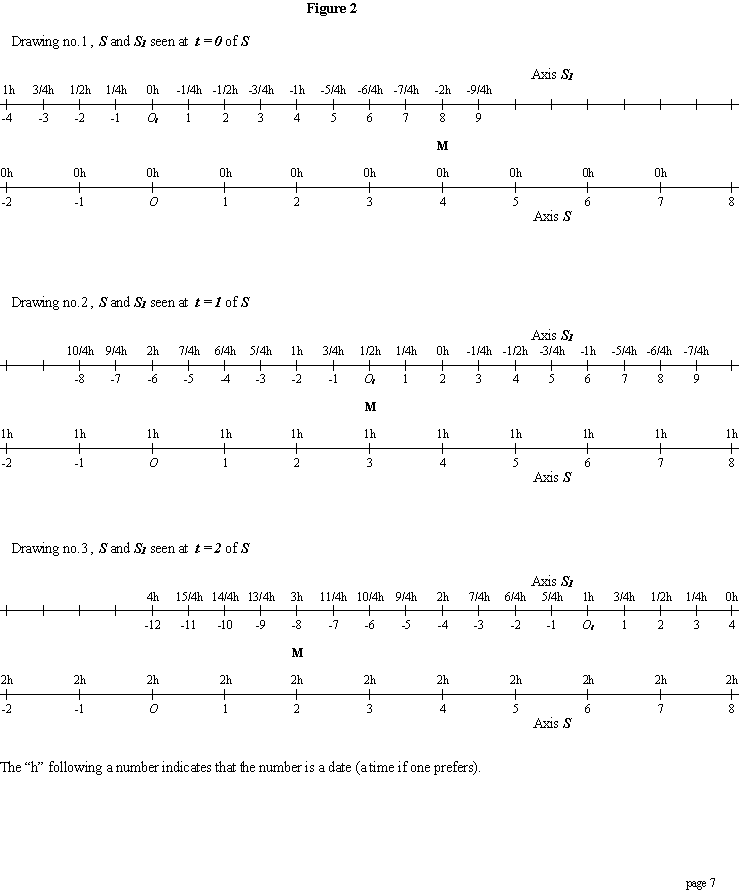Mechanical systems that verify  Lorentz' transformations.

1. System built (arbitrary appearance) .

Let a motionless Ox axis in a classical space time. This axis is noted S .
Let an O1x1 axis noted S1 , parallel to Ox , and in translation at speed v = 3  ,
relatively to Ox (in classical manner).

Axiomatically we take the unit of length  S1 to be half  the unit of S , and, on each point of S1
we place a clock , clocks that progress at the same speed twice as slow as those of S ,
and that are phased out, each one compared to the other, phasing out being -1/4 for a difference
of abscissa of 1 on S1 .
And moreover, the clock that is placed on O1 , the origin of S1 , indicates 0 at the date t = 0 of S .

More rapidly, on S1 we measure, abscissas and times as indicated on the drawings of figure 1.
The manner to measure times in S1 is in no way a surprise , just think of the terrestrial time zones.

The events taking place in Paris are dated by Parisian clocks, and those taking place in New York are dated
by the clocks of that town. When one says that an explosion happened at noon in New York ,
it is always implied that the hour is given by the clocks of that town. Similary in all other cities.

Let us return to our systems. Let E an event (an explosion, for example) that happens on the point of abscissa x of S ,
at the date t of S .
t is given by the clock of S that is found on the point of abscissa x of S , but has no importance because
S is a classical system whose all clocks are phased in.

The abscissa x1 measured in S1 of this event , as well as the date t1 given by the clock of S1 that is found
in the center of this event E (clock on the point of abscissa x1 of S1) when it happens ,

are given by :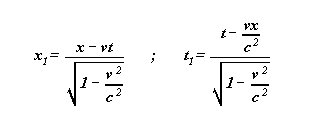With :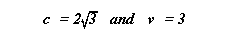One passes from S to S1 by Lorentz' transformations, which one can experiment with different events
on the drawings of the figure 1, or the figure 2 .

One notices that knowing x1 and t1 , one finds x and t with the two precedent formulas by replacing v by -v ,
therefore by the formulas :

Page 8We pass from S1 to S by Lorentz' formulas of same constant c .

Therefore we have two materially constructed systems, such that we pass from one to the
other by the Lorentz' transformations of same constant c .

The symmetry of these transformations does not in the least imply that S and S1
are analogous from a material viewpoint. In our drawings, systems are totally dissimilar,
and by seeing them, no one would think that they are equivalent,
and nevertheless, one passes from one system to the other by the same transformations.

We will see later that these two systems are not at all equivalent in the description of some
phenomena, especially moving bodies in translation in S at speeds greater than c , lead to some
(pseudo) paradoxes in S1 , entirely equivalent paradoxes to those obtained on the earth brought to
its time zones. A very fast plane that leaving Paris at noon (Parisian time) can arrive at eleven
o'clock in New York ( New York time) , and nobody would be shocked as one would be in relativity.

2° Natural system in classical mechanics.

One can wonder whether, in classical mechanics, there are systems that naturally obey
Lorentz' transformations, c being a constant attached to systems.

The reply is yes,they are, and it is even astonishing to see that they are very common.

Let a perfet elastic cord Ox , brought to an orthogonal referential Oxy system.

Any tranversal wave (of direction Oy ) on the cord is defined by two (any) functions f and g .

At any date t , the point of abscissa x of the cord has  y = f(ct -x) + g(ct + x)  for ordinate

c being a positive constant attached to the mechanical characteristics of the cord,
c is simply the speed of (transversal) waves along this cord.

y = f(ct -x) is on the cord, a wave propagating from left to right at  c speed,
y = g(ct + x) is on the cord, a wave propagating from right to left at the same speed c .
The sum of these two waves gives the state of the cord at any date t , and is the resultant wave.
The cord is oriented left to right.

Let t' and x' transformed of x and t by the Lorentz' formulas.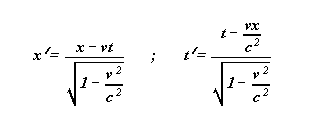As previously ,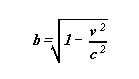Page 9

Let us calculate ct' - x' and ct' + x'

We find that :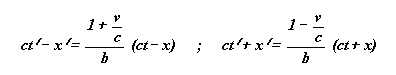Let us put :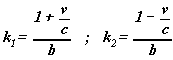k1 and k2 are two constants that do not depend either x or t , thus we find that

ct' - x' = k1 ( ct - x ) and that ct' + x' = k2 ( ct + x )

In the transversal perturbation y = f(ct -x) + g(ct + x) of the cord , replace x and t by x' and t' , we obtain :

y = f(ct' -x') + g(ct' + x') = f(k1 (ct -x)) + g(k2 (ct + x))

Which gives a new perturbation (which is entirely possible) of the same vibrant cord.

This new perturbation is the old one, moving at v speed along the Ox axis.
It is what is shown in the program (relauk.exe) accompagnying this text.

One can observe that if M is a motionless point of the starting perturbation , this same point is in translation
at speed v along the Ox axis , in the pertubation put at speed v .

Example : the transversal perturbation of the cord, defined by :    y = f(ct -x) - f(ct + x)  ,
admits the point of abscissa x = 0 as a motionless point.

In the perturbation put at speed v :       y = f(ct' -x') - f(ct' + x') = f(k1 (ct -x)) - f(k2 (ct + x)) ,
the point of abscissa x = vt  always has for ordinate y = 0 , and is easy to verify.

"Permanent" points of the starting perturbation , are converted to "permanent" points at speed v in the
perturbation put at speed v .

We now wonder :
What our clocks are on the cord ?
And what  they become when the perturbation is put at speed v ? .

On each point of the cord , in a motionless system , times are simply given by a stationary periodical
referential wave (or perturbation), the unit of time being proportional to the vibratory period.

The unit of length is defined by the same referential wave, the chosen unit being proportional to the distance
of two consecutive "permanent" points .

In any system put at speed v along Ox , time and length references are given by the referential wave put at speed v
along Ox , in conformity with Lorentz' transformations , as indicated above.

Let us specify this with an example: it is the example displayed when one starts the attached program.

Page 10

For a motionless system, we take as the stationary referential wave :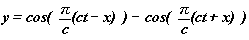(One can take c = 5 to be in conformity with the animation of the program).

The points of abscissa x = k c , k relative integer , are the only permanent points of this referential wave.
The unit of measure is the distance divided by c , between two consecutive permanent points .

On each point of the motionless system is a clock , a clock that advances one unit each time the wave
crosses under its feet the Ox axis.
(The vibratory period of this wave is T = 2 , Ox axis is crossed by the cord at every unit of time.)

When we put the system in motion at v speed along the Ox axis, times and distances in the system in
motion are given by the referential wave put at v speed, thus by the wave: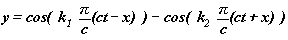(Constants k1 and k2 are defined above, in page 10.)

Each clock of the system in motion, always advances one unit each time the referential wave at speed v crosses
the Ox axis underneath. And the unit of length is obtained again by taking the distance between two consecutive
"permanent" points divided by c , always on the referential wave put at speed v.
The "permanent" points of the referential wave put at speed v , are in translation at speed v along Ox .

Now , "permanent" points are points of abscissas x = vt +bkc , k relative integer, b being defined above.
One sees that "permanent" points have drawn nearer according to multiplicative factor b .

A close observation of the animation of the attached program allows us to perfectly understand the above explanations.

This natural way of measure time and distance with the help of a referential wave, completely explains
Lorentz' transformations without exiting the framework of classical mechanics.

Complement : if Eo is the total energy , between two "permanent" points of the motionless "stationary wave" ,
the energy that takes into account the potential energy of the forces exerted by the wave on the extremities of the system
(forces exerted on the two permanent points), we find that the total energy Ev of the system at speed v is Ev = Eo/b
The démonstration is outside the limits of this elementary study.
The system behaves, relatively to the acceleration, as a mass of m = Ev / c2 .

The results obtained on a vibrating cord are generalized to three dimensional elastic mediums.

Experimental study of moving bodies travelling in S at speeds greater than c .

The aim of this short study is to underline some paradoxes that prove that S and S1 systems are not equivalent. S allows
to coherently describe the motion of a body moving at speeds greater than c , the description of the motion of this same
body becomes paradoxical (or incoherent) in S1 .

Page 11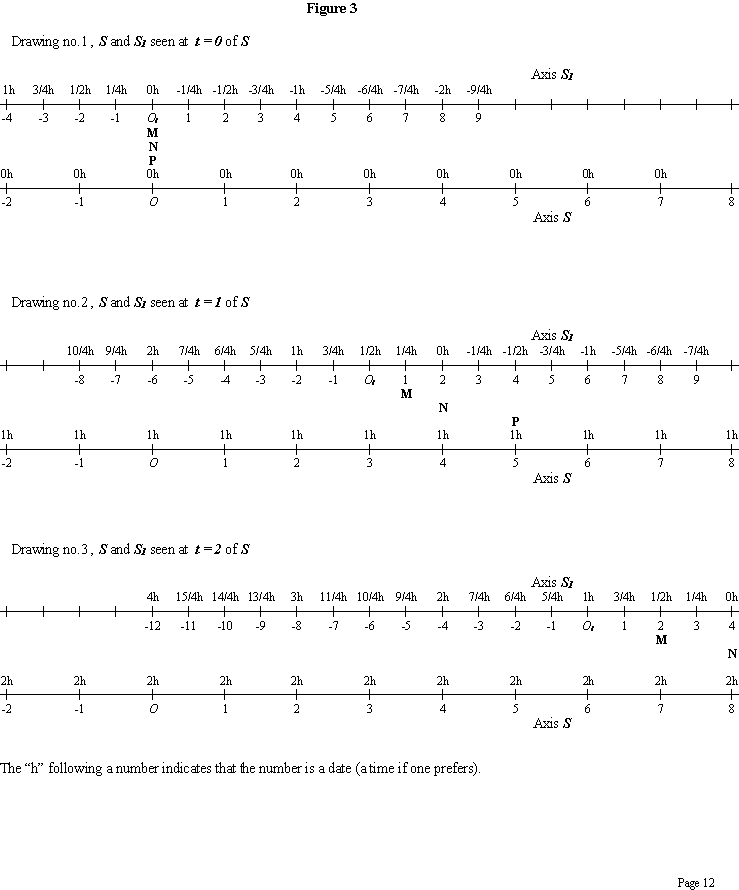Let us consider the drawings of figure 3.

Still with :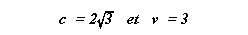Let M be a moving body in translation at speed v = 3.5 , relatively to S , its time équation in S being x = 3.5 t

At t = 0 of S , M is on x = 0 of S , it is perceived in S1 on the point of abscissa x1 = 0 ,
and the clock of S1 that is found on this point shows the date t1 = 0.

At the date t' = 2 of S , M is on x' = 7 of S, it is then perceived in S1 on the point of abscissa x'1 = 2 ,
and the clock of S1 that is found on this point shows the date t'1 = 1/2 .

The speed of M measured in the referential S1 is : (x'1- x1)/(t'1 - t1) = 2/0.5 = 4 .

The measure of the speed of M , relatively to S1 , gives a value greater than that obtained in S , both speeds
beeing greater than c .This result seems opposed to common sense which suggests for the speed of M , relatively to S1 ,
a smaller value than that obtained in S because M and S1 are going in the same direction (same sense).

This paradox is simply due to the way of measuring times and distances in S1 .

Now let N be another moving body in translation at speed 4 relatively to S
( the time equation of N in S being x = 4t ).
In S1 , the clocks of S1 that are found above N still show  0 , in other words, the moving body N crosses each clock
of S1 when this clock shows the date 0 . ( see the figure 3)
N is therefore, relatively to S1 , everywhere at the date 0 , the speed of  N , relatively to S1 , is infinite .
S1 is therefore a referential system in which it is impossible to descrbe the movement of N .
From this point of view , S and S1 are not equivalent.

Now, let the body P  moving at speed v = 5 , relatively to S
(the time equation of P , relatively to S , being taken equal to : x = 5 t ).
At the date t = 0 of S , P is on O origin of S , the coordinates of P in S1 are then
x1 = 0 and t1 = 0 .
At t' = 1 of S this same moving body P is found on the point of abscissa x' = 5 (in S).
As previously, with the help of figure 3 , we find that the coordinates of P in S1 are then
x'1 = 4 and t'1 = -1/2 .

In S1 , we have therefore : the starting date of P on O1 , 0 h .
the arrival date of P on x'1 = 4 , -1/2 h .
In S1 , the moving body P arrives 1/2 h before it has started.
Let us present this otherwise: in S1 , a marksman shoots a bullet from O1 at the date t1 = 0 ,and at speed
v = 5 , speed relative to S .

This bullet arrives on x'1 = 4 of S1 at the date t'1 = -1/2 h of S1 . The bullet , according to the measurement of S1 ,
arrives 1/2 h before it has started. In S1 , for some phenomena, the effect precedes the cause, or seems to precede the cause.
This paradox is analogous to those created by terrestrial time zone gaps.

Once again , S1 is inadequate for a coherent description of some phenomena. S and S1 are not equivalent.

This fact therefore allows to determine which of S or S1 is motionless.
The motionless system is that in which no paradox exists,  it is that in which the effect never precedes the cause.

It is always possible to add to S1 a Galilean system S'1 that follows S1
( clocks and units of S'1 are those of the classical mechanics , those of S ) .

The simultaneous existence of different types of referential systems is not in the least contradictory.
A system of Lorentz (relativistic system) , can always be doubled by a Galilean system in which it is easier to reason.

Page 13

The thoeretical study of speeds greater than c has been done in my book :
The relativity by the waving strings.
But what has been explained is enough to understand what happens.

The paradoxe of twins : this "paradox" is famous, I'll quickly mention it.

Let's take a system S2 that coincides with S1 (same as S1). S2 leaves S1 , then rejoins S1 by making the units coincide
(at the end of the experiment , the origin O2 of S2 is on O1 origin of S1 and the speed of S2 is equal to the speed of S1 ).

We notice then that the clocks of S2 are late compared with those of S1 , and this whatever the intermediate
accelerations of S2 , S1 continuing its uniform translation.
In the attachedprogram, the T key allows this experiment.
We can also draw different pictures as those of figures 1,2,3 with three systems to visualize this paradox.
This paradox is explained by Lorentz' formulas, without the help of any other theory.

Cabala Serge . 1975 , 1976, 1977 , 1980 , .... ,1999 , january 2000

P.S.

My mains works on this subject are:
- This study.
- Relativity by Waving Strings. (1975-76) classified in Archives Originales du CNRS (France) from 1977 (183 pages)
- Relativity by Elastic Mediums (1981, 185 pages)
- The Changes of variables that convert all functions of d'Alembertian equal to zero into functions of same property ,
with extension to Klein-Gordon equations (1988, 129 pages)

All these works have been accepted , and an ex-director of the French CNRS had underlined its quality.
They are neither taught not published on a large scale in order to preserve the traditional relativity.

It is to be regretted to see that science has been transformed into religion , by maintaining some unnecessary
and constraining dogmas , such as the principle of relativity and its limit speed.
The formulas of relativity being only classical mechanics formulas of elastic mediums, it is
unnecessary to wish to establish them from new principles, that arbitrarily limit their applications,
and introduce a degree of strange thought .

When one speaks about relativity, one always quotes the Michelson and Morley experiments,
done in 1881 and 1887 , on the detection of the wind of ether relative to the earth.

Let us reflect a bit, after having read what precedes and use the program on waves.
If electromagnetic fields are only particular "waves" of the ether considered as an elastic medium,
the only manner to put these "waves", or electromagnetic fields, in translation, is to use Lorentz' formulas,
and this in the framework of classical mechanics.
Atoms of matter are held together by "stationary" electromagnetics fields .
The setting in uniform lengthwise motion of a ruler of any material, puts the "stationary waves"
in motion , these "waves" are contracted in the direction of the motion and are phased out (see the program) ,
the ruler's atoms are compacted and the ruler is shortened.
The wind of ether becomes thus undetectable by this experiment.

The explanation of the negative result of this experiment, by the contraction of bodies in motion,
had already been given by Fitzgeral (1893), but the actual atomic constitution of the matter, not being known
(the atomistic constitution of the matter being even hardly denied by famous scientists) ,
this mechanical explanation seemed very difficult to admit.
One could not conceive, that materials of very different hardness at same velocity, can be contracted
in the same way.

The Michelson and Morley experiment , finely analyzed is in favor of the ether.

Page 14

This is near the analysis of the phenomenon of tides made in 17 th century, by Newton's detractors.

For these detractors: if the moon exerts an attraction on the earth , a sea swell must exist
in front of the moon and a hollow at the antipodes side.
But there are always two sea swells, one in front of the moon and another to lunar antipodes,
there is therefore no attraction.

The phenomenon of tides roughly explained by the universal attraction is opposite to reality,
but the universal attraction finely used with acceleration (what I do not do here) explains
perfectly why there are two swells.

It is similary with the Michelson and Morley experiment : roughly analized, it is in disfavor of the ether,
finely analized, it becomes one of the proofs.

I hope I have communicated the pleasure of at last a true Cartesian relativity.

Page 15